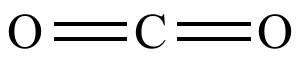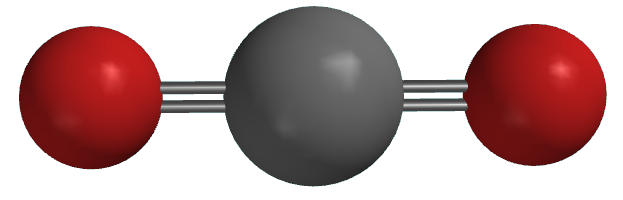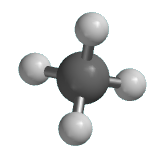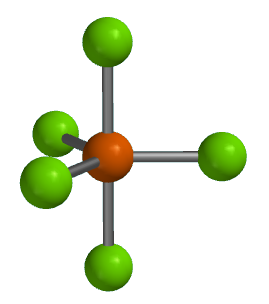Illustrated Glossary of Organic Chemistry

Steric number: The number of atoms, groups, or lone pairs (i.e., electron clouds) around a central atom. The steric number determines molecular geometry.Carbon dioxide
Steric number = 2
Geometry = linear

Boron trifluoride
Steric number = 3
Geometry = trigonal planar

Methane
Steric number = 4
Geometry = tetrahedral

Phosphorus pentachloride
Steric number = 5
Geometry = trigonal bipyramid

Sulfur hexafluoride
Steric number = 6
Geometry = octahedral